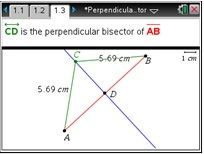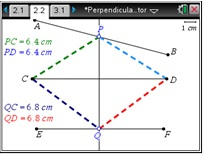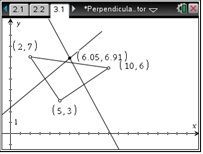# Activities

••• ##### Subject Area

• Math: Geometry: Triangles

• ##### Author9-12

15 Minutes

• ##### Device
•TI-Nspire™ CX/CX II
•TI-Nspire™ CX CAS/CX II CAS
• TI-Nspire™
• TI-Nspire™ CAS
• ##### Software

TI-Nspire™
TI-Nspire™ CAS

3.2

## Perpendicular Bisector

#### Activity Overview

In this activity, students will explore the perpendicular bisector theorem and discover that if a point is on the perpendicular bisector of a segment, then the point is equidistant from the endpoints. This is an introductory activity, where students will need to know how to change between pages, grab and move points, measure lengths, and construct the perpendicular bisector.

#### Key Steps

•Students will explore the distance from a point on the perpendicular bisector to the endpoints of a segment. They will measure AC and BC and then move point C around to find that the length of AC and BC is always the same.

•Students explore where a point lies if it is equidistant from the two endpoints. Students will notice that points P and Q both are on the perpendicular bisector of segment CD.

•Student will apply what they learned from Problem 1 and find the intersection of two perpendicular bisectors to find a point equidistant from three points.